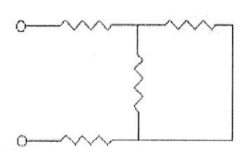# Problem: Each of the resistors in the circuit shown in the figure below has a resistance of 160.0 Ω. What is the equivalent resistance of the circuit? A) 160.0 Ω B) 400.0 Ω C) 480.0 Ω D) 640.0 Ω

🤓 Based on our data, we think this question is relevant for Professor Freedman's class at UCSB.

###### Problem Details

Each of the resistors in the circuit shown in the figure below has a resistance of 160.0 Ω. What is the equivalent resistance of the circuit?

A) 160.0 Ω

B) 400.0 Ω

C) 480.0 Ω

D) 640.0 Ω